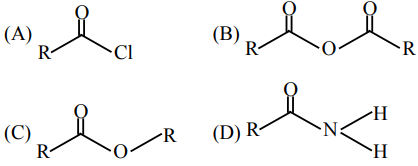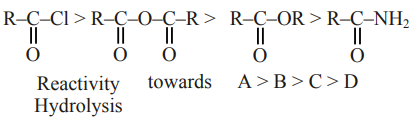# Solve this`
Question:The correct order of their reactivity towards hydrolysis at room temperature is :

1. $(\mathrm{A})>(\mathrm{B})>(\mathrm{C})>(\mathrm{D})$

2. $(\mathrm{D})>(\mathrm{A})>(\mathrm{B})>(\mathrm{C})$

3. $(\mathrm{D})>(\mathrm{B})>(\mathrm{A})>(\mathrm{C})$

4. $(\mathrm{A})>(\mathrm{C})>(\mathrm{B})>(\mathrm{D})$

Correct Option: 1,

Solution: